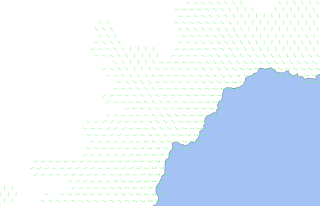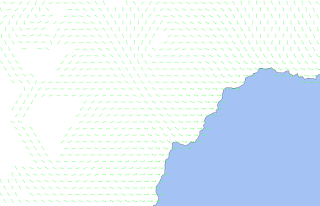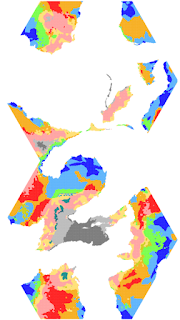## November 26, 2018

### Wind VI: Wind Pun

Getting the wind model to work is the easy part.

Figuring out what parameters to use is the hard part.

One issue that I ran into last time is the fact that if wind starts going in the same direction from a bunch of sources, simple vector addition can add up fast.

That's because I've been treating my wind collisions as elastic collisions. There are two major kinds: elastic and inelastic. In an elastic collision, no energy is lost to heat, etc. So two (or more) winds enter a hex, one wind leaves. The following formula is used:
$m_0 v_0 + m_1 v_1 + \cdots + m_n v_n = m_f v_f$
This is essentially what we've been doing, in two dimensions, treating all masses as equal (even the final mass). Because I'm ignoring masses, this isn't really a physically correct description of an elastic collision.

The other option is inelastic. I think this is better, because we can think of the input winds "sticking" together into a new mass of air. This is not how fluids really mix, however, but it will be good enough. This formula is (ignoring masses again):
$v_f = {v_0 + v_1 + \cdots + v_n \over n}$
Which is simply the average.

So let's try a few combos of parameters. $g$ is the ratio of decrease between each successive push, and $z$ is the slope change (in feet) at which the wind is deflected by 45 degrees.g=0.7, z=5000, elasticg=0.7, z=5000, inelasticg=0.7, z=2500, inelasticg=0.8, z=2500, inelasticg=0.9, z=2500, inelasticg=0.99, z=2500, inelastic
Overall, the inelastic collision seems much more accurate. I also need to take a look at the downhill slope speed increase, which is not set for these simulations. The wind is still modulating into bands of 60 degrees, even with Gaussian blurring. Scott has trouble with straight bands, I have trouble in an additional dimension!

Also, I need to develop some better representation for topography. The heightmap is huge overkill here, but its difficult to see the effect of altitude when height is not shown at all. Something for a rainy day (of which there are many upcoming).

## November 21, 2018

### Wind V: Clearer Winds

As often happens, I'll publish an initial post on some subject, then add bells and whistles on to my code, then post the "finished" product with a few quips, and expect the audience to have insight into the chaos of my workflow.

So I've decided to put the wind model down on blog-paper, at least to make it clearer to myself and others. I was inspired by Here Dragons Abound, who also revisited his wind model and arrived at the same method that I did (just with better pictures)! So this post is essentially the same, just with some different words and pictures, for my own reference.

Getting the initial wind values at the coast was the easy part, really. But what to do once I have them?

Ideally, I could use some kind of iterative finite element method to solve for the flow across the surface. But that's pretty complicated and if I can find something simpler, I will.

There are a few things to keep in mind as we create this model.

• Wind slows as it drags across the ground
• Wind picks up speed when going downhill and slows uphill
• Wind is turned aside by mountains
So there are (in the most simple representation) 3 parameters that can be tuned. I'll call them $g$ for ground drag, $s$ for slope change (where $\Delta$ can represent the different in height of the hexes), and $\theta(\rho, \Delta)$ for the change in direction by a mountain (or hill, etc), where $\rho$ is the sensitivity of the wind.

Let's begin with a single wind vector.

The basic method of propagation is along the vector projection of the direction between the two hexes. I refer you to this diagram from the post on tectonics. We are interested in the $d_{\perp f}$ vector.

Projecting the vectors:

One downside of this approach is that eventually, every initial vector gets binned into one of the 6 hex directions, so the wind map becomes much more West Marches-esque, with strictly defined angles in increments of 60 degrees. But let's propagate each of these three resultants.

Next, we add the vectors (simply adding the $x$ and $y$ components) for E and B.

And of course, E and B will project onto D, and so on. But I'll ignore that for now.

So what about mountains, or other obstacles? How much is it affected? Let us assume that A represents a high mountain at 10,000 ft, and that the other hexes are 1 ft. We can then define $\theta$ in terms of $\Delta$ as:
$\theta = {90 \exp\left(\rho\Delta\right) \over 90 + \left(\exp\left(\rho\Delta\right) - 1\right)}$

The value $\rho={\ln(89) \over 5000}$ indicates that the wind is turned aside 45$^\circ$ at a slope of 5000 ft. So for our Mountain A, it will be 89$^\circ$. I have no idea what number will work best here. The relative direction of change is to the right in the northern hemisphere, and to the left in the southern hemisphere.

And so it continues (I haven't actually propagated A out fully).

As far as picking up speed goes, I have $s = g$ if $\Delta < 0$ else $1$. But I want to do some tweaking of this anyway (I also see a lot of stuff that I want to update/clean up, just from going through this exercise). I've been using $g = 0.946$ after my initial experiments.

So the final model for the effect of wind from Hex 0 to Hex 1 (where $h_{01}$ represents the vector from 0 to 1):
$w_{01} = g \cdot s \cdot {w_0 \cdot h_{01} \over |h_{01}|^2} h_{01} + \angle \theta(\rho, \Delta)$
I guess that looks kinda complicated. If you can't wow them with facts, dazzle them with bullshit.

There is also a choice to be made in the algorithm for propagating vectors. Either we can view this as an expanding frontier, where the next set of hexes to be solved is defined by where the vectors in the current frontier are pointing (projection is only ever positive for 3 hexes at most), or we can fully propagate each vector from each source (which are all coastal hexes) and then average them at the end. Right now I'm using source-propagation since its a little faster, but I'd like to take another look at frontier-propagation.

I'll save the good stuff (the actual application on the map) for another post.

## November 20, 2018

### Elevation XI: Uplift Revisited

One of the most important inputs to the erosion model is the tectonic uplift. I've tried a few things with this in the past, and now I'm back again for another crack at it.

The basic idea behind a hex's uplift value is its distance to convergent, transform, and divergent faults. It's difficult to compress this all into a single number, so I played around with the values until I arrived at this, where i and max_i represent the maximum distance from the C/D/T faults:

uplift = (
0.5 * c/max_c +
0.1 * t/max_d -
0.4 * d/max_t + 0.399
)


So every hex will have a relative amount of uplift on a scale of 0 to 1. I do rescale this later so it goes from 0.1 to 1: no uplift means problems like sub-sea elevation terrain that I don't want to have to deal with (thanks, New Orleans).Fault map; yellow is the highest uplift, blue is highest divergence, and white is a conjunction of all three
This number can then be fed into the erosion model to determine how much the land is rising while water is trying to eat it away. Usually, the tectonic plate wins.

So this gives us the first version of the uplift model. There are a few surprises, where I expected to see higher values, but it turns out that these are in proximity to divergent boundaries which reduce the overall number.

But this is too simple (of course). Plates don't collide like this: they ripple. There's a good approach here on one solution to this. I'll take a different tack, simply using a screening mask to to modify the values. Yet another optimization step! But this is what I will use initially, after much mucking around with different values, where $u_0$ is the initial uplift, $u_f$ is the final uplift, and $a$ is some constant.
$u_f = \left(u_0 + {2 \sin(a u_0)\over a} + {\cos(a u_0^2) \over a}\right)^3$

Looks ok. Just too smooth. So we'll salt it a bit with some simplex noise:

There's still a significant problem as it relates to the overall terrain, though. There's really only one major mountain range. Everything else is at least half that value. Either 1) this is ok, and just an acceptable outcome of the inputs or 2) I can use gamma correction to modify the map.

But I'll tackle that later.

## November 14, 2018

### Resources XVI: Manganese and Nickel Miningnickelmagnanese

If we want good steel, we need manganese and nickel. Strictly speaking, these are ingredients of wrought iron, but I'm sticking them into steel. The Spartans may have lucked into manganese-rich iron ores, producing superior weapons.

Thankfully, nickel is easy. It's found in a form of limonite, which I've already categorized as an iron ore. So a tiny bit of limonite will yield nickel, and therefore it's not a Stage 0 raw resource.

Manganese is not terribly difficult to research either. It comes from pyrolusite (found, like everything else, with chalcopyrite). In the interest of fantasy-style names, I'll call it umber instead (which is a rich brown pigment made of crushed pyrolusite). In fact, I wonder what might be some more fantastical names for hematite, etc. Good subject for a future post.

Umber has an occurrence per hex of 2%, but this is improved by the presence of goethite, hematite, mountainous terrain, or boggy areas.

## November 12, 2018

### Resources XV: Revisiting Metals in Demoland

Now that we have data on all the metals we need, I'm going to revisit the resource distribution in Demoland. From here, we can gradually build up society as it might have expanded in this kingdom.

This map shows potential resources in each hex, not necessarily the exploited ones. I've also left off agricultural references, because the map tends to get pretty cluttered, even with just a few symbols. The symbol reference can be found here. I've grayed out the symbols on purpose. Once we being actually placing them (again), they'll be more visible to indicate their exploitation.

Maps like this can be helpful for certain types of campaigns. Knowing what materials are where can inform an ambitious party searching for a source of wealth, or a place to found a town and be king of their own country. At least, until Rich Neighbor gets jealous...

## November 9, 2018

### Resources XIV: Placing Copper Ore

Chalcopyrite has some relation to both gold and silver, and so it could be considered a lynchpin of this system. But we will also consider the other copper ores here as well.

I've found a really good resource at Mindat.org, which gives the number of mines around the world for different minerals. I can plug this into my formula and get a percentage occurrence for my own world.
• Chalcopyrite: 27,901 deposits. 16.9%
• Malachite: 12,759 deposits, 7.8%
• Azurite: 5,593 deposits, 3.4%
Not bad. Azurite and malachite are linked, and chalcopyrite is linked with volcanic areas and coal seams.

## November 7, 2018

### Resources XIII: Placing Silver Ore: Redux

I made an error in the previous post. To enshrine my mistake, I'll fix it here.

I forgot to multiply by the hex area factor. If I do so, I get an occurrence of 700%! That implies that there are 7 deposits of silver in each hex. That has to be wrong, otherwise silver would be essentially worthless. So I made a bad assumption.

Instead, I will use the Mindat method (discussed in the future copper post). This yields:
${5,484\textrm{ deposits}\over 57,000,000\textrm{ mi}^2}\cdot {347\textrm{ mi}^2 \over 1 \textrm{ hex}} = 3.3\%$
That's a bit more reasonable. Again, keep in mind that 347 square miles is a massive area, and that's just one hex!

### Resources XII: Placing Silver Ore

Silver's crust abundance is 0.0000079%, so about 2000x more common than gold. Let's use the numbers already generated for gold:
${2000\cdot580\textrm{ deposits}\over 57,000,000\textrm{ mi}^2}\cdot 347\textrm{ mi}^2 = 2\%$
For now, I'll ignore galena and pretend we're mining pure silver (galena, remember, is only about 2% silver). Just like with gold, we see an opportunity to increase the occurrence alongside chalcopyrite, which is quickly becoming my favorite mineral. So, to get the placement for gold and silver down, I need to tackle copper ores.

Edit: In which I correct a mistake.

## November 6, 2018

### Desirability II: Where Do All the People Go?

I've been getting way behind on posting, largely because of my struggles with getting the climates to fall out just right. I know, I know, there's a fair bit of ad hoc going on here. But I've had some trouble in the past with my climates swinging directly from harsh rainforest to harsh deserts. I've got to figure out some methods to smooth that out.

First of all, I can lower the temperature model. It's not just the rain - although that hurts too. Temperature is an odd bird, especially trying to go from this chart on Cartographer's Guild to some actual numbers that I can use for my model. I may need to step back to those isotherms and see if I can build up the coherent model from that. I've been trying several different math functions to try and get smooth interpolation, but I haven't found one that works.Koppen map
I want to see more green here. The real world has a lot of green (which is the C climate type, really good for crops and such). Some tweaking improved this. However, there's still very little D (boreal), denoted by turquoise. This isn't really a problem, exactly, but I wonder how much of this is due to the terrain/climate system and how much is due to the errors of the model.

However, I can take another look at my "desirability" maps. I still have some tweaking to do to make the right recipe, and then do it again for all the races I want to include! Here is where the climate distribution shows its ugly belly.The greener, the better
It seems at first glance that there's a lot of empty space. Everyone wants coastline, of course, but no one wants to be in the desert (humans, anyway; I like the idea of desert orcs).